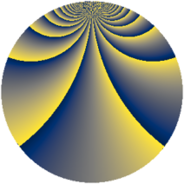# Properties

 Label 900.3.bvLevel $900$ Weight $3$ Character orbit 900.bv Rep. character $\chi_{900}(13,\cdot)$ Character field $\Q(\zeta_{60})$ Dimension $960$ Sturm bound $540$

# Related objects

## Defining parameters

 Level: $$N$$ $$=$$ $$900 = 2^{2} \cdot 3^{2} \cdot 5^{2}$$ Weight: $$k$$ $$=$$ $$3$$ Character orbit: $$[\chi]$$ $$=$$ 900.bv (of order $$60$$ and degree $$16$$) Character conductor: $$\operatorname{cond}(\chi)$$ $$=$$ $$225$$ Character field: $$\Q(\zeta_{60})$$ Sturm bound: $$540$$

## Dimensions

The following table gives the dimensions of various subspaces of $$M_{3}(900, [\chi])$$.

Total New Old
Modular forms 5856 960 4896
Cusp forms 5664 960 4704
Eisenstein series 192 0 192

## Trace form

 $$960q + 2q^{3} + O(q^{10})$$ $$960q + 2q^{3} - 22q^{15} - 36q^{17} + 12q^{23} - 24q^{25} - 46q^{27} + 14q^{33} - 72q^{35} + 84q^{37} + 400q^{39} + 210q^{45} + 78q^{47} + 312q^{53} - 132q^{55} - 302q^{57} + 150q^{59} - 868q^{63} - 768q^{65} + 78q^{67} - 350q^{69} + 120q^{71} + 354q^{75} - 140q^{81} + 1128q^{83} - 96q^{85} + 478q^{87} + 1500q^{89} - 174q^{93} + 168q^{95} + 90q^{97} + O(q^{100})$$

## Decomposition of $$S_{3}^{\mathrm{new}}(900, [\chi])$$ into newform subspaces

The newforms in this space have not yet been added to the LMFDB.

## Decomposition of $$S_{3}^{\mathrm{old}}(900, [\chi])$$ into lower level spaces

$$S_{3}^{\mathrm{old}}(900, [\chi]) \cong$$ $$S_{3}^{\mathrm{new}}(225, [\chi])$$$$^{\oplus 3}$$$$\oplus$$$$S_{3}^{\mathrm{new}}(450, [\chi])$$$$^{\oplus 2}$$